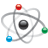## How to configure HVI0 function in MC9s12vr64?

cancel
Showing results for
Search instead for
Did you mean:

## How to configure HVI0 function in MC9s12vr64?

268 ViewsContributor II

After i configure the HVI0 function as following code, i can not get the right result from ATD result register ATDDR0.

Please help to finger out mistakes in my code. Thanks very much!

Of course , if you can give some suggestion, that will be best.

PTL init:

//********PTL INIT *********

DIENL |=  0x02U;  //PTIL0 is set to  analog input

//& PTIL1   are set to Digital Input for Down Switch(PTIIL1)

//PTIL2 & PTIL3 are reserved ;

PTAL = 0x08U;

PIRL = 0x03U;

PPSL = 0x00U;    // Falling edge selected for Active interrupt edge

PIEL = 0x00U;     //  Interrupt is disabled;  PIFL is interrupt flag

//*************************************

ATD init:

/* PIRL: PIRL3=0,PIRL2=0,PIRL1=0,PIRL0=1 */

PIRL = 0x01U;

/* Initialization of the ADC module */

/* ATDCTL0: WRAP3=1,WRAP2=1,WRAP1=1,WRAP0=1 */

ATDCTL0 = 0x0FU;

/* ATDCTL1: ETRIGSEL=0,SRES1=0,SRES0=1,SMP_DIS=0,ETRIGCH3=1,ETRIGCH2=1,ETRIGCH1=1,ETRIGCH0=1 */

ATDCTL1 = 0x2FU;

/* ATDCTL3: DJM=0,S8C=0,S4C=0,S2C=1,S1C=0,FIFO=0,FRZ1=0,FRZ0=0 */

ATDCTL3 = 0x90U;

/* ATDCTL4: SMP2=0,SMP1=0,SMP0=0,PRS4=0,PRS3=0,PRS2=1,PRS1=0,PRS0=1 */

ATDCTL4 = 0x05U;

/* ATDCTL5: SC=0,SCAN=0,MULT=0,CD=0,CC=0,CB=0,CA=0 */

ATDCTL5 = 0x00U;

/* ATDCTL2: AFFC=0,ETRIGLE=0,ETRIGP=0,ETRIGE=0,ASCIE=0,ACMPIE=0 */

ATDCTL2 = 0x00U;

Test codes in main.c:

ATDCTL5 = 0x8bU;

i look the result register _ATDDR0_Word in data1 windows of IDE.

It is always feedback result around 500;

Thanks very much!

Labels (1)
• ### General

3 Replies
76 ViewsContributor II

Thanks  Iggi &  Radek!!!

76 ViewsNXP Employee

Hi Juejie,

Here is the example SW for your purpose. Also attached is another one demonstrating wake up from Stop mode with HVI.

Hope it helps.

iggi

76 ViewsNXP Employee

Hi Juejie,

Unfortunately, you didn’t specify some important details – like which voltage you applied to the HVI0 pin or bus clock.

You selected ATDCTL4_PRS=5. In that case, bus clock will be divided by 12. Therefore I suppose that your bus clock is 24 or 25MHz. Correct?

I do not understood your command ATDCTL5 = 0x8bU;.

The highest bit is reserved. I suppose that you want to set SC bit - Special Channel Conversion Bit.

In that case, you probably measure AN5 channel instead HVI0 pin. The command should be ATDCTL5 = 0x4BU;

BTW: you selected the sequence length value (S8C, S4C, S2C, S1C) = 2. It means that you should get two results in ATDDR0 and ATDDR1 registers.

BTW: you selected RatioL_HVI =2 in your configuration. In that case, the maximum voltage at pin must be below 10V.

I hope it helps you.

Have a great day,
Radek

-----------------------------------------------------------------------------------------------------------------------
Note: If this post answers your question, please click the Correct Answer button. Thank you!
-----------------------------------------------------------------------------------------------------------------------